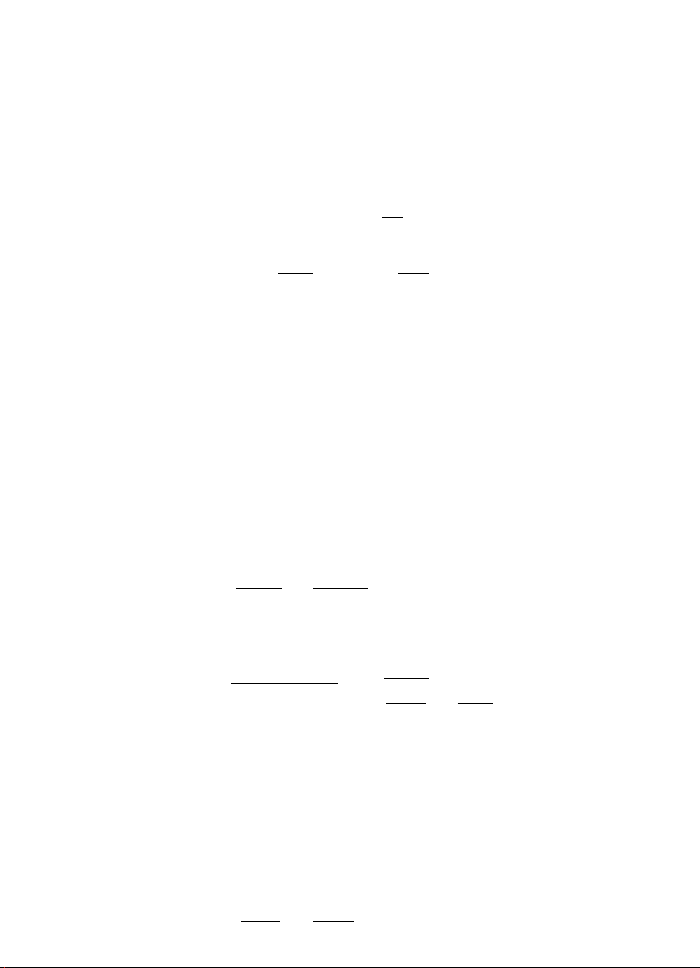3Linear models

IB Statistics3.6 Simple linear regression
We can apply our results to the case of simple linear regression. We have
Y
i
= a
+ b(x
i
¯x) + ε
i
,
where ¯x =
P
x
i
/n and ε
i
are iid N(0, σ
2
) for i = 1, ··· , n.
Then we have
ˆa
=
¯
Y N
a
,
σ
2
n
ˆ
b =
S
xY
S
xx
N
b,
σ
2
S
xx
ˆ
Y
i
= ˆa
+
ˆ
b(x
i
¯x)
X
i
(Y
i
ˆ
Y
i
)
2
σ
2
χ
2
n2
,
and a
,
ˆ
b) and ˆσ
2
= RSS/n are independent, as we have previously shown.
Note that
ˆσ
2
is obtained by dividing RSS by
n
, and is the maximum likelihood
estimator. On the other hand,
˜σ
is obtained by dividing RSS by
n p
, and is
an unbiased estimator.
Example. Using the oxygen/time example, we have seen that
˜σ
2
=
n p
=
67968
24 2
= 3089 = 55.6
2
.
So the standard error of
ˆ
β is
SE(
ˆ
b) =
q
˜σ
2
(X
T
X)
1
22
=
r
3089
S
xx
=
55.6
28.0
= 1.99.
So a 95% interval for b has end points
ˆ
b ± SE(
ˆ
b) × t
np
(0.025) = 12.9 ± 1.99 t
22
(0.025) = (17.0, 8.8),
using the fact that t
22
(0.025) = 2.07.
Note that this interval does not contain 0. So if we want to carry out a size
0
.
05 test of
H
0
:
b
= 0 (they are uncorrelated) vs
H
1
:
b
= 0 (they are correlated),
the test statistic would be
ˆ
b
SE(
ˆ
b)
=
12.9
1.99
=
6
.
48. Then we reject
H
0
because
this is less than t
22
(0.025) = 2.07.